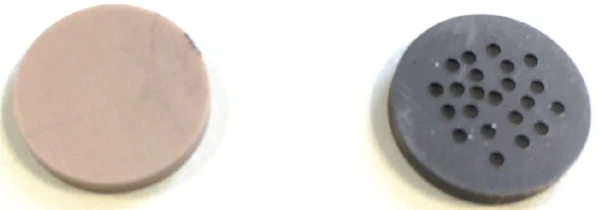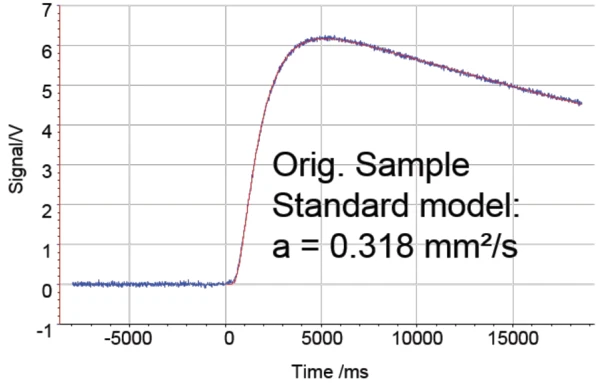# Penetration Model in the NETZSCH LFA Software − Porous Materials Finally Handled Properly!

#### Introduction

Software models that consider the influence of the shape and surface of specimens are becoming more and more important for the precise determination of thermophysical properties (TPP) such as Thermal DiffusivityThermal diffusivity (a with the unit mm2/s) is a material-specific property for characterizing unsteady heat conduction. This value describes how quickly a material reacts to a change in temperature.thermal diffusivity (a), Thermal ConductivityThermal conductivity (λ with the unit W/(m•K)) describes the transport of energy – in the form of heat – through a body of mass as the result of a temperature gradient (see fig. 1). According to the second law of thermodynamics, heat always flows in the direction of the lower temperature.thermal conductivity (λ) and Specific Heat Capacity (cp)Heat capacity is a material-specific physical quantity, determined by the amount of heat supplied to specimen, divided by the resulting temperature increase. The specific heat capacity is related to a unit mass of the specimen.specific heat capacity (cp). For this reason, in recent years NETZSCH has been committed to continually improving existing LFA (laser flash analysis) models and to developing new calculation models, corrections and mathematical operations considering heat loss in combination with pulse correction, radiation, multilayer systems, in-plane tests, baseline corrections, etc.

This application note presents the Penetration model based on McMasters . It is suitable for measurements on materials with rough surfaces and on extremely porous materials.

#### Porous Materials Are a Challenge – But Not for the Penetration Model

In standard flash measurements, the front face of the specimen absorbs the total energy. A thermal wave will then travel through the specimen’s thickness before reaching the rear face (figure 1). For porous materials, NETZSCH has now introduced the Penetration model (figure 2) that includes the following considerations:

• Absorption of the pulse energy is no longer limited to the front face
• Absorption is extended over a thin layer into the specimen's thickness
• Absorption layers can be handled as the mean free path in the material

Consideration of these aspects results in an exponentially decaying initial temperature distribution within the specimen. Application of this approach, which accounts for the porosity of the material, results in improved accuracy and precision for the Thermal DiffusivityThermal diffusivity (a with the unit mm2/s) is a material-specific property for characterizing unsteady heat conduction. This value describes how quickly a material reacts to a change in temperature.thermal diffusivity, Thermal ConductivityThermal conductivity (λ with the unit W/(m•K)) describes the transport of energy – in the form of heat – through a body of mass as the result of a temperature gradient (see fig. 1). According to the second law of thermodynamics, heat always flows in the direction of the lower temperature.thermal conductivity and Specific Heat Capacity (cp)Heat capacity is a material-specific physical quantity, determined by the amount of heat supplied to specimen, divided by the resulting temperature increase. The specific heat capacity is related to a unit mass of the specimen.specific heat capacity values determined.

#### Measurement Conditions

For testing the suitability of the Penetration model, two filled polymers made of the same type but of different shapes were measured. One measurement was carried out on a specimen with a surface, which was covered with boreholes of 0.5 mm in diameter. For comparison reasons, a second measurement was carried out on the original specimen with a smooth surface (figure 3). The Thermal DiffusivityThermal diffusivity (a with the unit mm2/s) is a material-specific property for characterizing unsteady heat conduction. This value describes how quickly a material reacts to a change in temperature.thermal diffusivity was determined on specimen dimensions of 12.7 mm in thickness and 1.96 mm in diameter at room temperature.3) Filled polymer disc on the left, polymer disc with boreholes on the right

#### Measurement Results

Figures 4 and 5 depict the measurement on the sample with boreholes. In figure 4, the model fit of the detector rise signal (red curve) is obtained by using the standard model by Cowan . The green circle indicates the area of deviations between the fit and the measurement curve (blue). With this – obviously insufficient – model fit, the Thermal DiffusivityThermal diffusivity (a with the unit mm2/s) is a material-specific property for characterizing unsteady heat conduction. This value describes how quickly a material reacts to a change in temperature.thermal diffusivity is calculated at 0.753 mm2/s. The calculation based on the Penetration model yields a Thermal DiffusivityThermal diffusivity (a with the unit mm2/s) is a material-specific property for characterizing unsteady heat conduction. This value describes how quickly a material reacts to a change in temperature.thermal diffusivity of 0.626 mm2/s, which is nearly 17% lower in value (figure 5).

Figure 6 shows the rise of the detector signal from the measurement on the original filled polymer disc with smooth surface. Using the standard Cowan model here for determination of the Thermal DiffusivityThermal diffusivity (a with the unit mm2/s) is a material-specific property for characterizing unsteady heat conduction. This value describes how quickly a material reacts to a change in temperature.thermal diffusivity yields nearly the same measurement results as were obtained with the Penetration model for the specimen with boreholes (figure 5). The deviation amounts to approximately 3%. This proves that the calculation of the Thermal DiffusivityThermal diffusivity (a with the unit mm2/s) is a material-specific property for characterizing unsteady heat conduction. This value describes how quickly a material reacts to a change in temperature.thermal diffusivity based on the Penetration model yields correct results.6) Measurement on the original specimen without boreholes, fit of the detector rise curve obtained using the standard Cowan model

#### Conclusion

Along with the various classical models (e.g., Cowan 5 /10, Parker, improved Cape-Lehman, etc.), the NETZSCH LFA Proteus® software includes many different calculation models, corrections and mathematical operations. The Penetration model is specifically suitable for porous materials and materials with a rough surface. This special feature of the LFA Proteus® software involves the penetration of the light flash into the specimen beyond the actual heated surface. It accounts for the specimen’s porosity, which causes much of the light flash energy to be deposited inside the specimen. This means the Penetration model considers for absorption of the pulse energy over a thin layer into the specimen thickness. Measurements on samples of the same specimen but with very different surface structures (smooth vs. porous), confirm the correctness of the Penetration model.

#### Literature

1. 
McMasters, Beck, Dinwiddie, Wang (1999): “Accounting for Penetration of Laser Heating in Flash Thermal Diffusivity Experiments”, Journal of Heat Transfer, 121,15-21
2. 
Cowan, Robert D.; Journal of Applied Physics, Vol. 34, Number 4 (Part 1), April 1963